# Monopulse Antennas

A monopulse antenna is one method of realizing a tracking radar. The word "monopulse" implies that with a single pulse, the antenna can gather angle information, as opposed to spewing out multiple narrow-beam pulses in different directions and looking for the maximum return.

The monopulse uses four antennas (or quadrants of a single antenna). They can be horns, or sections of a flat plate array of radiators, or even subarrays of an active electrically scanned antenna (AESA) phased array. The elements are all steered together mechanically (on a gimbal) or electrically (using phase shifters in the case of AESA). The target is illuminated by all four quadrants equally. A comparator network is used to "calculate" four return signals. The sum signal has the same pattern in receive as transmit, a broad beam with highest gain at boresight; the sum signal is used to track target distance and perhaps velocity. The elevation difference signal is formed by subtracting the two upper quadrants from the two lower quadrants, and is used to calculate the target's position relative to the horizon. The azimuth difference signal is formed by subtracting the left quadrants from the right quadrants and is used to calculate the target's position to the left or right. A fourth signal, called the "Q difference" is the diagonal difference of the quadrants; this signal is often left to rot an a termination, so the typical monopulse receiver needs only three channels. Sometimes only a two-channel receiver is used, as the two difference signals are multiplexed into one with a switching arrangement.

Here's a block diagram of a monopulse antenna, including the comparator network. The blue squares represent 180 degree hybrid couplers (such as rat-races). You can follow the simple arithmetic as the analog signals are added and subtracted to form the four receiver channels.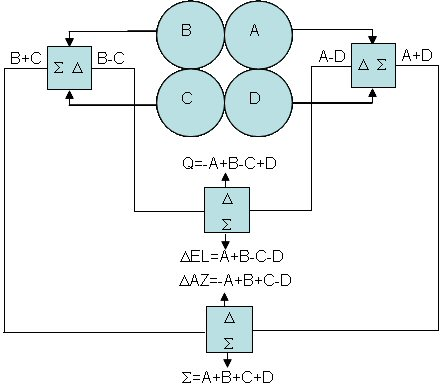According to convention, elevation angle is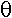. What happens when the target is not along the boresight of the radar? Let's look at the geometry of target with respect to angle, for a simple monopulse where only two antenna elements (horn perhaps) are used. The target is some distance L from the upper quadrants of the monopulse antenna. It is slightly farther away (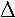l) from the lower quadrants, which varies as the sine of the angle and the distance separating the antennas.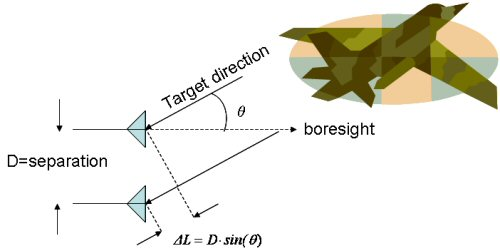Let's look at the sum and difference outputs versus elevation anglefrom boresight. In this case we will space the antennas one wavelength apart, and did the math using a combination of Eagleware Genesys (to model the tranfer function of the comparator network) and Excel (to model the horn). Here we have assumed an omni-directional antenna (no gain pattern).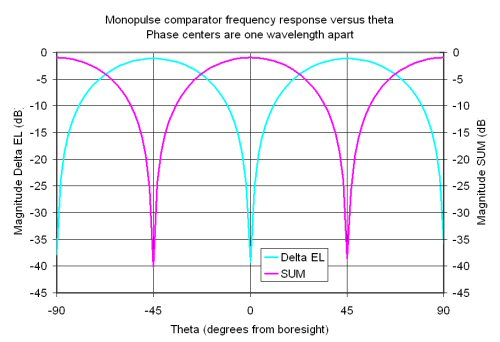Now we "overlay" the horn antenna pattern to see the pattern you'd observe when you test this puppy in an anechoic chamber: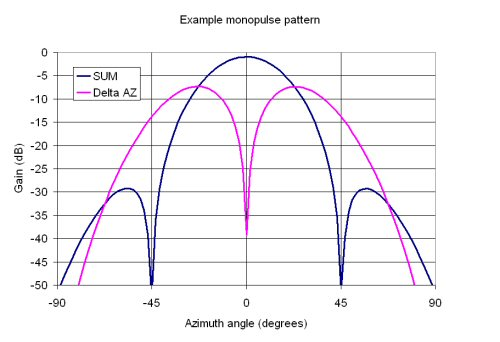The null depth is a measure of how well the signals cancel each other. In our example we have achieved 38 dB (the difference between sum and delta in dB).

Cool stuff!

Author : Unknown Editor